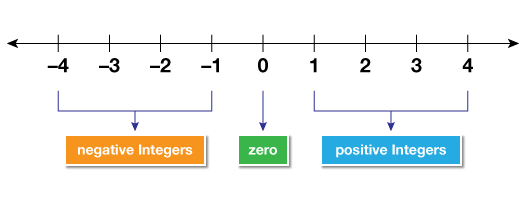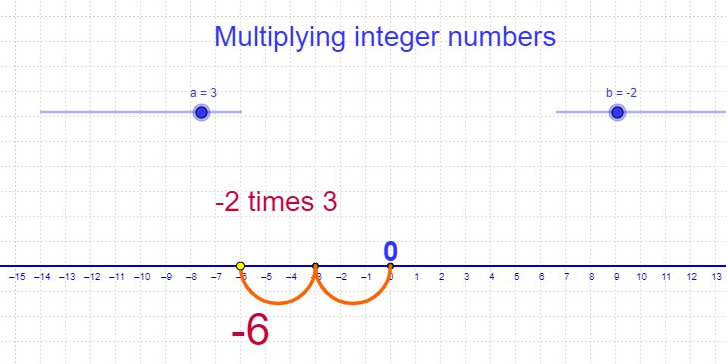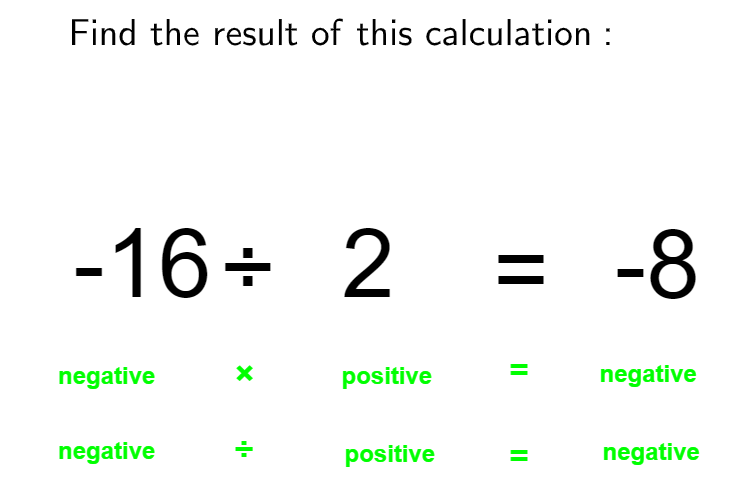# Multiplication and Division of Integers

Go back to  'Integers'

## Introduction to Multiplication and Division of Integers

Multiplication is a process of repetitive addition. Division, on the other hand, is nothing but a process of repetitive subtraction. But what happens when there are negative numbers involved? And how do they have an impact in the real world?

## The big idea behind multiplication and division of integers

To simplify the multiplication of whole or natural numbers, it is nothing but a process in which a number is added to itself for a particular number of times.

The process of division of whole or natural numbers is splitting up and distributing parts of a whole in equal groups. With division, you start with a larger number and break it down multiple times till you are left with the smallest number possible

### What are integers and their representation on the number line

An integer by definition is a whole number that can be either positive, negative or zero (but cannot be a fraction). Integers are represented by Z and are written as;

$$\text{Z} = \text{{…, –3, –2, –1, 0, 1, 2, 3, …}}$$

Integers cannot be $$-1.25,$$ $${2 \over 3},$$ $$-589.332$$As long as you keep track of the signs, multiplication and division of integers is the same.

When you multiply two integers the same sign;

Positive x positive = positive = 2 x 5 = 10

When you multiply two integers with two negative signs;

Negative x Negative = Positive = –2 x –3 = 6

When you multiply two integers with one negative sign and one positive sign;

Negative x positive = negative = –2 x 5 = –10When you divide two integers with the same sign;

Positive ÷ positive = positive 16 ÷ 8 = 2

When you divide two integers with two negative signs;

Negative ÷ negative = positive = –16 ÷ –8 = 2

When you divide two integers with one negative sign and one positive sign;

Negative ÷ positive = negative = –16 ÷ 8 = –2To sum it all up to make everything easy, the two most important things to remember whether you are multiplying or dividing integers;

1. When the signs are different, the answer is always negative.
2. When the signs are the same, the answer is always positive.

### Multiplication as a problem

Your child has Rs.10/- in their piggy bank. They owe 3 of their friends Rs.2/- each. How much in total do they owe their friends and what is left over in their piggy bank after the distribution?

### The solution

Your child owes Rs.2/- to 3 friends; (–2) + (–2) + (–2)  OR  3 X –2

Since they owe their friends money, it is represented as a decrease from the total amount. They owe them Rs.2, hence representing it as –2. According to the number line, we make a move from 0 towards the left side into the negative numbers. We move to the left by 2 digits, 3 times.

Hence 3 x (–2) = –6In the end, they owe his friends a total of Rs 6. And the total amount they has left over in their piggy bank is 10 – 6 = Rs.4/-.

### Division as a problem

A girl loves to play video games, but each time her character fell down a cliff, she lost points. At the end of the game, she had lost 40 points and had fallen 5 times. How many points did she lose each time she fell?

### The solution:

The girl lost 40 points, hence –40. She fell 5 times.
–40 ÷ 5 = –8

Each time the girl fell, she lost 8 points.

## Problems faced during multiplication and division of integers

Most students are confused when there are more than 2 integers with different signs that have to be either multiplied or divided. The confusion of what the resultant sign is is the big question. To make it easier the rules of multiplication and division of integers is of utmost importance. Working the equation from left to right, applying the rules to each sign makes sure that mistakes will not be made. For example;

### Division;

(–20) ÷ (–5) ÷ (–2) =?

### The solution;

Step 1: (–20 ÷ –5) ÷ (–2)
Step 2: (10) ÷ (–2)
10 is positive since 2 negative ÷ negative = positive
Step 3: –5
Since positive ÷ negative = negative
Hence (–20) ÷ (–5) ÷ (–2) = –5

### Multiplication;

(–6) x (–4) x (3) =?

### The solution;

Step 1: (–6 x –4) x (3)
Step 2: 24 x 3
24 is positive since negative X negative is positive
Step 3: 72
Hence (–6) x (–4) x (3) = 72